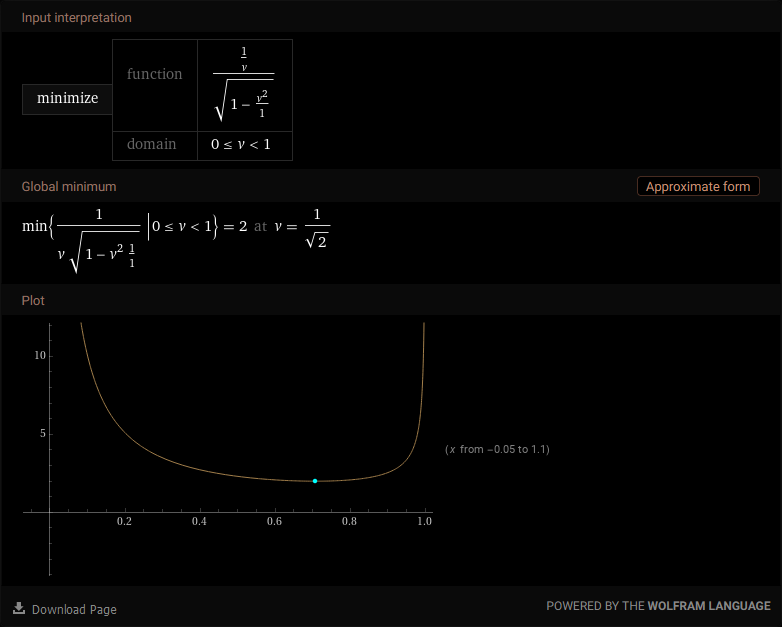# Fastest Space Travel, Smallest Time Dilation

Note: A bug in WordPress or the LaTeX plugin used to display equations on this blog has broken the formatting at the end of this post, and I can’t figure out why or how to fix it.

You’re the captain of a medical ship responding to a distress call from the next solar system. You need to arrive as fast as possible. How fast do you go?

It sounds like a ridiculous question until you think about time dilation due to moving at relativistic speeds. If you want to skip straight to the answer, it’s ≈0.707c. If you travel any slower, it takes longer to get there (duh).. but the interesting part is that if you travel any faster, you also arrive later. While the trip time for you is purely based on how fast you move, the arrival time is based on how fast you move relative to your destination.

Welcome to relativity. A simple equation relates how much time passes between an observer at rest (your destination) and an observer in motion (you):

$t = t0 / \sqrt{1 – v^2 / c^2}$

t is the time at the destination, while t0 is the time that passes for you. You need to know how fast to go, so I’ve rearranged this equation to solve for a velocity based on known times:

$v = \sqrt{ c^2t^2-c^2t_0 } / t$

Of course, we don’t know what those times are either. It’s a bit of a conundrum (which kept me from solving this problem for over 2 years). This next part? I solved it at 3 am a few days ago, and then forgot what logical leap got me here. I have no idea why this works, I just know that it does:

$v \in [0,1)$ $t_0 = 1 / v$ $t = t_0 / \sqrt{ 1 – t_0^2 }$

The first line defines the valid range of velocities (anything from stationary to lightspeed, except for lightspeed itself). The second and third lines confuse the hell out of me. No idea how I figured out that your time is 1 / v. I know it has something to do with using multiples of c as velocity values so that I can replace occurrences of c with 1. It also has something to do with assuming 1 second passes for the stationary observer (your destination, t), allowing it to also be replaced with 1 as well.

Somehow these assumptions led to an equation with only t0 on the right side, and t on the left – what needs to be minimized! Again, we need to solve for velocity, so we substitute t0 with 1 / v:

$t = v \sqrt{1 – v^2}$

This is the part where I cheated and had Wolfram Alpha find the minimum for me:If you travel as fast as possible, your arrival time is only double your trip time. Of course, because of the acceleration and deceleration times, you will never hit exactly 2x time dilation over the total trip – but you don’t want to anyhow, as the only way to hit that value would be to go even faster – and guarantee you arrive even later than you otherwise would.

This site uses Akismet to reduce spam. Learn how your comment data is processed.